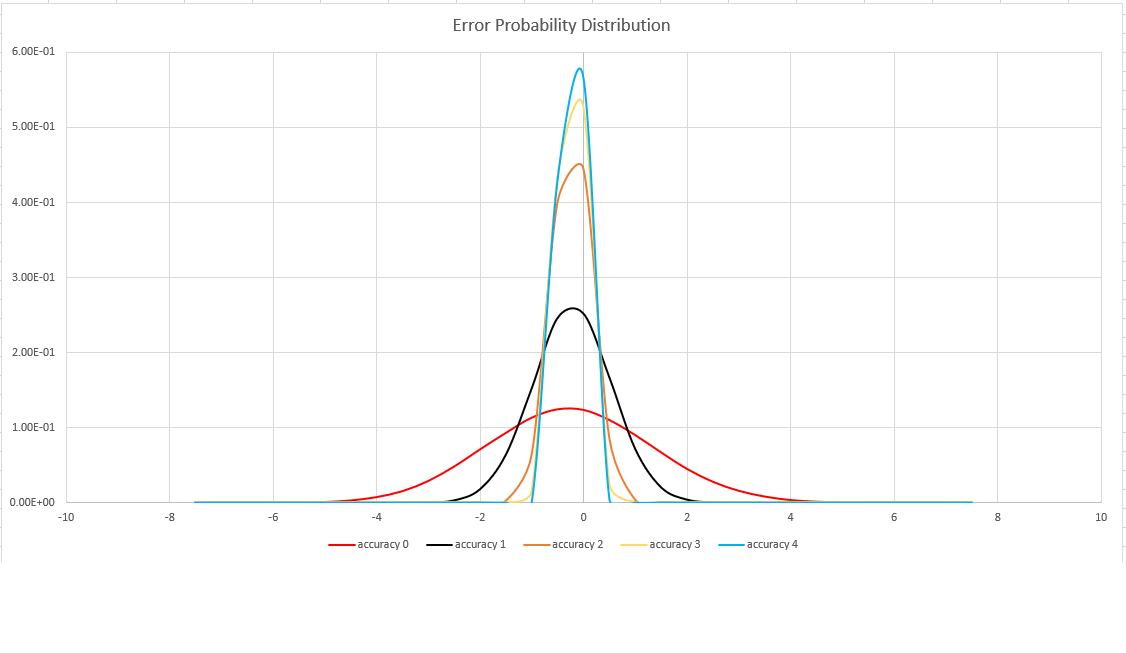# dcountif() (aggregation function)

Calculates an estimate of the number of distinct values of Expr of rows for which Predicate evaluates to `true`.

Note

This function is used in conjunction with the summarize operator.

## Syntax

`dcountif` `(`Expr, Predicate, [`,` Accuracy]`)`

## Arguments

Name Type Required Description
Expr string Expression that will be used for aggregation calculation.
Predicate string Expression that will be used to filter rows.
Accuracy int Controls the balance between speed and accuracy. If unspecified, the default value is `1`. See Estimation accuracy for supported values.

## Returns

Returns an estimate of the number of distinct values of Expr of rows for which Predicate evaluates to `true` in the group.

Tip

`dcountif()` may return an error in cases where all, or none of the rows pass the `Predicate` expression.

## Example

This example shows how many types of fatal storm events happened in each state.

``````StormEvents
| summarize DifferentFatalEvents=dcountif(EventType,(DeathsDirect + DeathsIndirect)>0) by State
| where DifferentFatalEvents > 0
| order by DifferentFatalEvents
``````

Results

The results table shown includes only the first 10 rows.

State DifferentFatalEvents
CALIFORNIA 12
TEXAS 12
OKLAHOMA 10
ILLINOIS 9
KANSAS 9
NEW YORK 9
NEW JERSEY 7
WASHINGTON 7
MICHIGAN 7
MISSOURI 7
... ...

## Estimation accuracy

This function uses a variant of the HyperLogLog (HLL) algorithm, which does a stochastic estimation of set cardinality. The algorithm provides a "knob" that can be used to balance accuracy and execution time per memory size:

Accuracy Error (%) Entry count
0 1.6 212
1 0.8 214
2 0.4 216
3 0.28 217
4 0.2 218

Note

The "entry count" column is the number of 1-byte counters in the HLL implementation.

The algorithm includes some provisions for doing a perfect count (zero error), if the set cardinality is small enough:

• When the accuracy level is `1`, 1000 values are returned
• When the accuracy level is `2`, 8000 values are returned

The error bound is probabilistic, not a theoretical bound. The value is the standard deviation of error distribution (the sigma), and 99.7% of the estimations will have a relative error of under 3 x sigma.

The following image shows the probability distribution function of the relative estimation error, in percentages, for all supported accuracy settings: